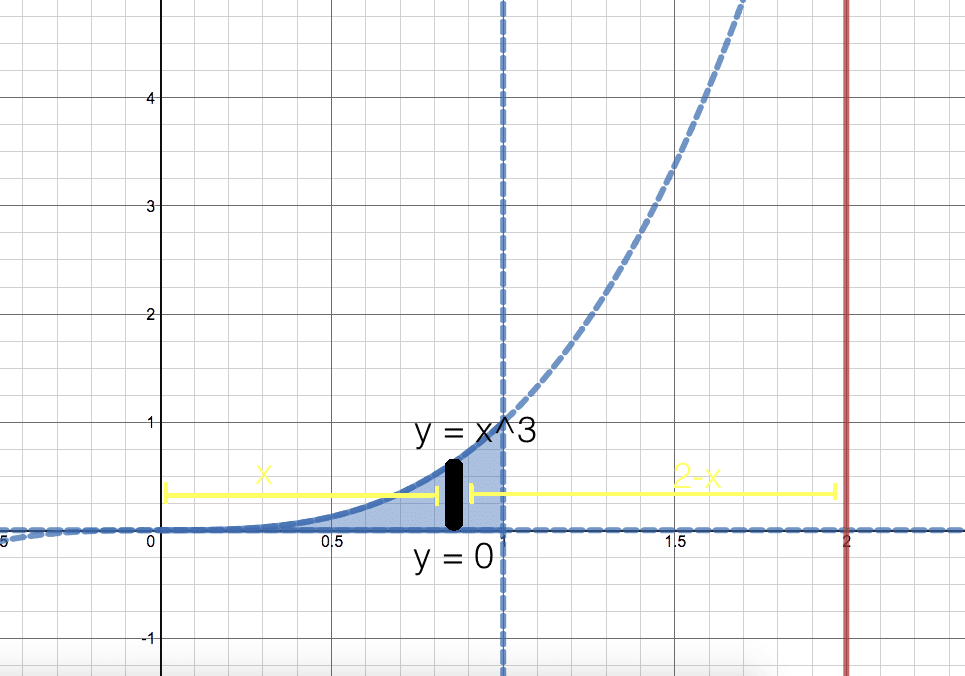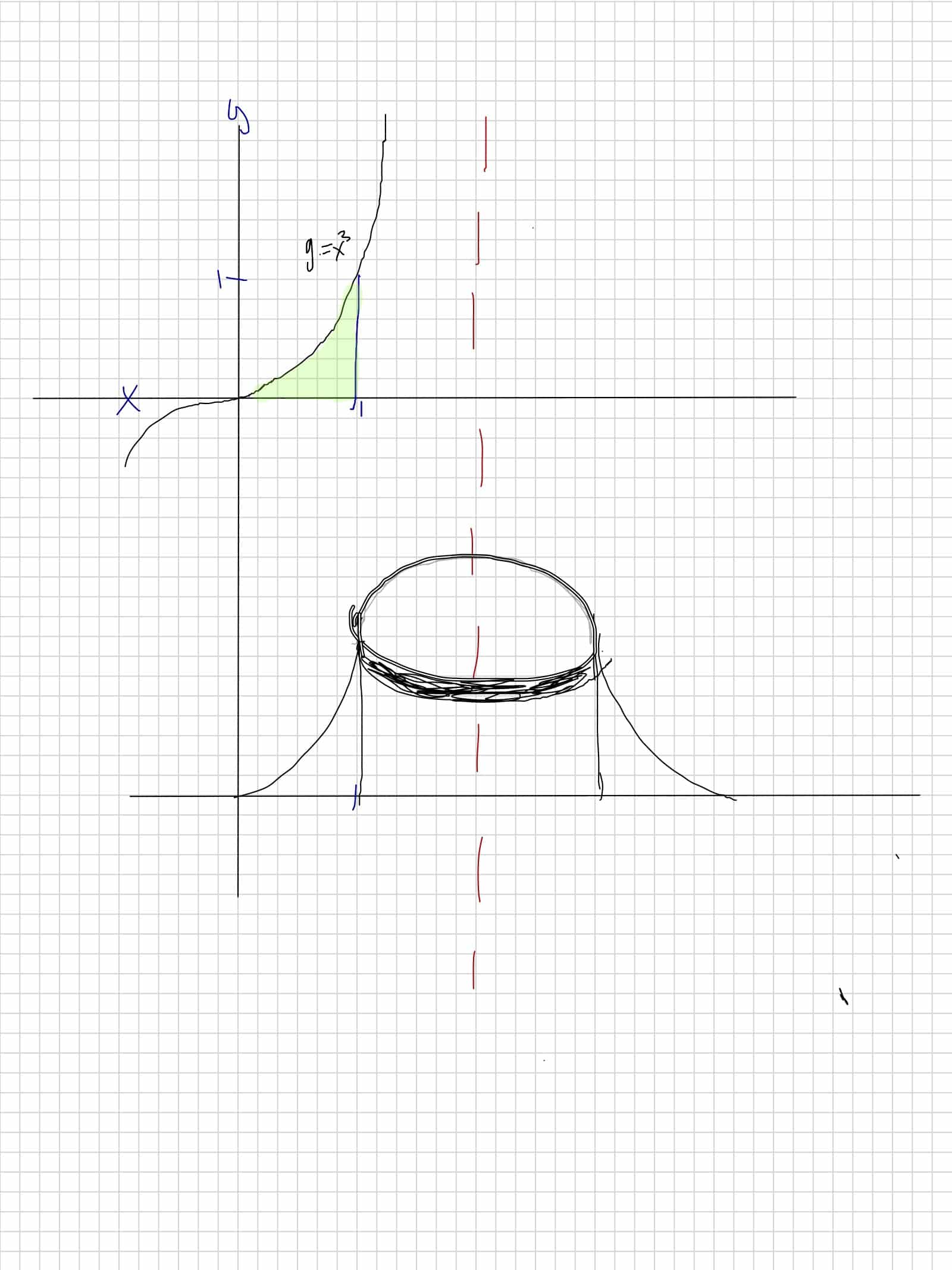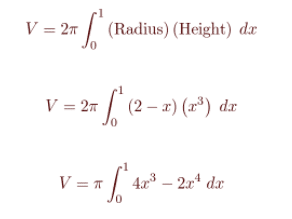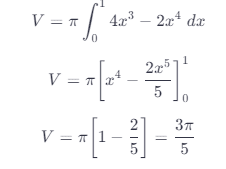# Y=x^3 Y=0 X=1 About X=2

We thoroughly check each answer to a question to provide you with the most correct answers. Found a mistake? Let us know about it through the REPORT button at the bottom of the page.

Find the volume of the solid obtained by rotating the region bounded by the given curves about the specified line. Sketch the region, the solid, and a typical disk or washer.

y = x3, y = 0, x = 1; about x = 2When we rotate a thin vertical strip as shown in the figure, about the line x = 2.

We get a cylindrical shell with a radius 2 – x

The height of the cylindrical shell is x^3To find the complete volume, we will integrate from 0 to 1,